# Computing Variance, Standard Deviation, and Other Statistical Functions

Problem

You want to compute one or more of the common statistics such as variance, standard deviation, skew, and kurtosis of a sequence of numbers.

Solution

You can use the accumulate function from the header to compute many meaningful statistical functions beyond simply the sum by passing custom function objects. Example 11-9 shows how to compute several important statistical functions, using accumulate.

Example 11-9. Statistical functions

```#include
#include
#include
#include
#include
#include

using namespace std;

template
T nthPower(T x) {
T ret = x;
for (int i=1; i < N; ++i) {
ret *= x;
}
return ret;
}

template
struct SumDiffNthPower {
SumDiffNthPower(T x) : mean_(x) { };
T operator( )(T sum, T current) {
return sum + nthPower(current - mean_);
}
T mean_;
};

template
T nthMoment(Iter_T first, Iter_T last, T mean) {
size_t cnt = distance(first, last);
return accumulate(first, last, T( ), SumDiffNthPower(mean)) / cnt;
}

template
T computeVariance(Iter_T first, Iter_T last, T mean) {
return nthMoment(first, last, mean);
}

template
T computeStdDev(Iter_T first, Iter_T last, T mean) {
return sqrt(computeVariance(first, last, mean));
}

template
T computeSkew(Iter_T begin, Iter_T end, T mean) {
T m3 = nthMoment(begin, end, mean);
T m2 = nthMoment(begin, end, mean);
return m3 / (m2 * sqrt(m2));
}

template
T computeKurtosisExcess(Iter_T begin, Iter_T end, T mean) {
T m4 = nthMoment(begin, end, mean);
T m2 = nthMoment(begin, end, mean);
return m4 / (m2 * m2) - 3;
}

template
void computeStats(Iter_T first, Iter_T last, T& sum, T& mean,
T& var, T& std_dev, T& skew, T& kurt)
{
size_t cnt = distance(first, last);
sum = accumulate(first, last, T( ));
mean = sum / cnt;
var = computeVariance(first, last, mean);
std_dev = sqrt(var);
skew = computeSkew(first, last, mean);
kurt = computeKurtosisExcess(first, last, mean);
}

int main( )
{
vector v;
v.push_back(2);
v.push_back(4);
v.push_back(8);
v.push_back(10);
v.push_back(99);
v.push_back(1);
double sum, mean, var, dev, skew, kurt;
computeStats(v.begin( ), v.end( ), sum, mean, var, dev, skew, kurt);
cout << "count = " << v.size( ) << "
";
cout << "sum = " << sum << "
";
cout << "mean = " << mean << "
";
cout << "variance = " << var << "
";
cout << "standard deviation = " << dev << "
";
cout << "skew = " << skew << "
";
cout << "kurtosis excess = " << kurt << "
";
cout << endl;
}```

The program in Example 11-9 produces the following output:

```count = 6
sum = 124
mean = 20.6667
variance = 1237.22
standard deviation = 35.1742
skew = 1.75664
kurtosis excess = 1.14171```

Discussion

Some of the most important statistical functions (e.g., variance, standard deviation, skew, and kurtosis) are defined in terms of standardized sample moments about the mean. The precise definitions of statistical functions vary somewhat from text to text. This text uses the unbiased definitions of the statistical functions shown in Table 11-1.

Table 11-1. Definitions of statistical functions

Statistical function

Equation

nth central moment (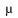n)VarianceStandard deviation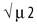Skew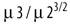Kurtosis excess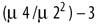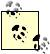A moment is a characterization of a sequence of numbers. In other words, it is a way to describe a set of number mathematically. Moments form the basis of several important statistical functions, such as the variance, standard deviation, skew, and kurtosis. A central moment is a moment that is computed about the mean as opposed to about the origin. A sample moment is a moment that is computed from a discrete set of numbers instead of a function. A standardized moment is a moment divided by a power of the standard deviation (the standard deviation is the square root of the second moment).

The simplest way to code the statistical functions is to define them all in terms of moments. Since there are several different moments used, each one accepting a constant integer value, I pass the constant as a template parameter. This allows the compiler to potentially generate more efficient code because the integer is known at compile time.

The moment function is defined using the mathematical summation operator . Whenever you think of the summation operation you should think of the accumulate function from the header. The accumulate function has two forms: one accumulates using operator+ and the other uses an accumulator functor that you need to provide. Your accumulator functor will accept two values: the accumulated value so far, and the value at a specific position in the sequence.

Example 11-10 illustrates how accumulate works by showing how the user supplied functor is called repeatedly for each element in a series.

Example 11-10. Sample implementation of accumulate

```template
Iter_T accumulate(Iter_T begin, Iter_T end, Value_T value, BinOp_T op) {
while (begin != end) {
value = op(value, *begin++)
}
return value;
}```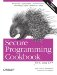Secure Programming Cookbook for C and C++: Recipes for Cryptography, Authentication, Input Validation & More
ISBN: 0596003943
EAN: 2147483647
Year: 2006
Pages: 241